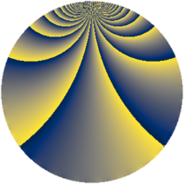# Properties

 Label 1792.2.qLevel $1792$ Weight $2$ Character orbit 1792.q Rep. character $\chi_{1792}(383,\cdot)$ Character field $\Q(\zeta_{6})$ Dimension $120$ Sturm bound $512$

# Learn more

## Defining parameters

 Level: $$N$$ $$=$$ $$1792 = 2^{8} \cdot 7$$ Weight: $$k$$ $$=$$ $$2$$ Character orbit: $$[\chi]$$ $$=$$ 1792.q (of order $$6$$ and degree $$2$$) Character conductor: $$\operatorname{cond}(\chi)$$ $$=$$ $$56$$ Character field: $$\Q(\zeta_{6})$$ Sturm bound: $$512$$

## Dimensions

The following table gives the dimensions of various subspaces of $$M_{2}(1792, [\chi])$$.

Total New Old
Modular forms 560 136 424
Cusp forms 464 120 344
Eisenstein series 96 16 80

## Trace form

 $$120q + 56q^{9} + O(q^{10})$$ $$120q + 56q^{9} - 12q^{17} - 40q^{25} - 12q^{33} - 40q^{49} - 72q^{57} + 16q^{65} + 108q^{73} - 44q^{81} + 12q^{89} + O(q^{100})$$

## Decomposition of $$S_{2}^{\mathrm{new}}(1792, [\chi])$$ into newform subspaces

The newforms in this space have not yet been added to the LMFDB.

## Decomposition of $$S_{2}^{\mathrm{old}}(1792, [\chi])$$ into lower level spaces

$$S_{2}^{\mathrm{old}}(1792, [\chi]) \cong$$ $$S_{2}^{\mathrm{new}}(56, [\chi])$$$$^{\oplus 6}$$$$\oplus$$$$S_{2}^{\mathrm{new}}(224, [\chi])$$$$^{\oplus 4}$$$$\oplus$$$$S_{2}^{\mathrm{new}}(448, [\chi])$$$$^{\oplus 3}$$$$\oplus$$$$S_{2}^{\mathrm{new}}(896, [\chi])$$$$^{\oplus 2}$$